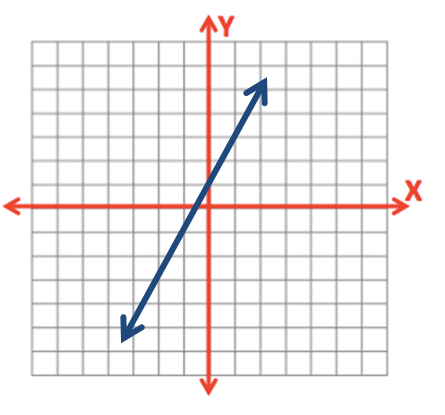# 0.1.3 - Basic Linear Equations

0.1.3 - Basic Linear Equations

You may recall from an algebra class that the formula for a straight line is $y=mx+b$ where $m$ is the slope and $b$ is the y-intercept. The x-axis is the horizontal axis and the y-axis is the vertical axis.The y-intercept is where the line crosses the y-axis.  The slope is a measure of change in y over change in x, sometimes written as $\frac{\Delta y}{\Delta x}$ where $\Delta$ ("delta") means change.

## Example

$y=2x+1$

Here, the y-intercept is 1 and the slope is 2.  This line will cross the y-axis at the point (0, 1). From there, for every one increase in $x$, $y$ will increase by 2. In other words, for every one unit we move to the right, we will move up 2.In statistics, the y-intercept is typically written before the slope. The equation for the line above would be written as $y=1+2x$.

Different notation may also be used:

$\widehat y = a + bx$, where $\widehat y$ is the predicted value of y, $a$ is the y-intercept and $b$ is the slope.

$\widehat y = b_0 + b_1 x$, where $\widehat y$ is the predicted value of y, $b_0$ is the y-intercept, and $b_1$ is the slope.

  Link ↥ Has Tooltip/Popover Toggleable Visibility